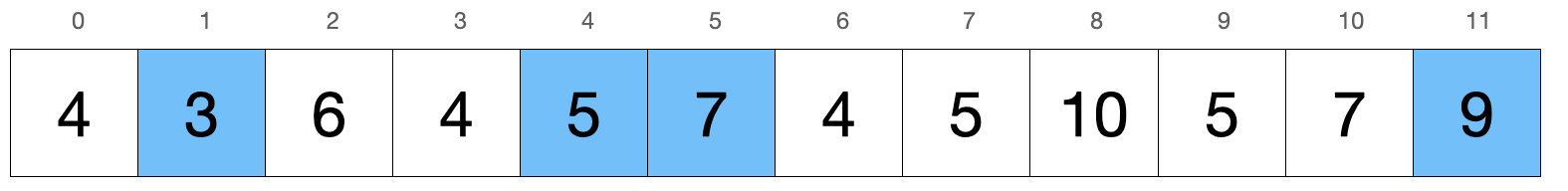# LeetCode 1218. Longest Arithmetic Subsequence of Given Difference Solution

Given an integer array arr and an integer difference, return the length of the longest subsequence in arr which is an arithmetic sequence such that the difference between adjacent elements in the subsequence equals difference.

A subsequence is a sequence that can be derived from arr by deleting some or no elements without changing the order of the remaining elements.

Example 1:

Input: arr = [1,2,3,4], difference = 1
Output: $4$
Explanation: The longest arithmetic subsequence is [1,2,3,4].

Example 2:

Input: arr = [1,3,5,7], difference = 1
Output: $1$
Explanation: The longest arithmetic subsequence is any single element.

Example 3:

Input: arr = [1,5,7,8,5,3,4,2,1], difference = -2
Output: $4$
Explanation: The longest arithmetic subsequence is [7,5,3,1].

Constraints:

• $1\leq$ arr.length $\leq 10^5$
• $-10^4\leq$ arr[i], difference $\leq 10^4$

## Brute Force

For this problem, let's think of building the subsequence one integer at a time. If our subsequence is currently ending with the integer $k$, our next integer will have to be $k$ $+$ difference. Since subsequences must follow the relative order of the original array, we want to pick the next closest value of $k$ $+$ difference and append it to our subsequence. We can also observe that appending the closest value of $k$ $+$ difference will give us more options for the next addition to our subsequence.

To find the longest subsequence however, we can try all possible starting positions for our subsequence and construct it greedily with the method mentioned above. Out of all subsequences, we'll return the length of the longest one.

### Example

For this example, we'll start the sequence at index $1$ and difference is $2$.Our subsequence starts with $3$ and we're looking for $3$ $+$ difference $=5$. It appears at indices $4,7,$ and $9$. We want the next closest position so we'll pick the $5$ at index $4$. We apply the same idea to then pick $7$ at index $5$ and finally $9$ at index $11$.

Let $N$ represent arr.length.

Since building the subsequence takes $\mathcal{O}(N)$ and we build $\mathcal{O}(N)$ subsequences (one for each starting position), this algorithm runs in $\mathcal{O}(N^2)$.

## Full Solution

For a faster solution, we'll use dynamic programming. We know that to extend subsequence ending with $k$, we'll find the next closest element with value $k$ $+$ difference and add it into our subsequence. We can also think of this idea from the other direction. To find the subsequence ending with $k$ $+$ difference at some position, we'll look for the previous closest element $k$. Then, we'll take the longest subsequence ending with that specific element $k$ and append $k$ $+$ difference to obtain our desired subsequence. This idea uses solutions from sub-problems to solve a larger problem, which is essentially dynamic programming.

Let dp[i] represent the length of the longest subsequence with the last element at index $i$.

Let j represent the previous closest index of the value arr[i] - difference. If j exists, then dp[i] = dp[j] + 1 since we take the longest subsequence ending at index j and append arr[i] to it. Otherwise, our subsequence is just arr[i] itself so dp[i] = 1.

We can maintain the previous closest index of integers with a hashmap. The hashmap will use a key for the integer and the value will be the closest index of that integer. Everytime we process dp[i] for some index $i$, we'll update arr[i] into our hashmap. Our final answer is the maximum value among all values in dp.

### Time Complexity

Since we take $\mathcal{O}(1)$ to calculate dp[i] for one index $i$, our time complexity is $\mathcal{O}(N)$ since dp has a length of $\mathcal{O}(N)$.

Time Complexity: $\mathcal{O}(N)$

### Space Complexity

Our dp array and hashmap both use $\mathcal{O}(N)$ memory so our space complexity is $\mathcal{O}(N)$.

Space Complexity: $\mathcal{O}(N)$

## C++ Solution

1class Solution {
2   public:
3    int longestSubsequence(vector<int>& arr, int difference) {
4        int n = arr.size();
5        unordered_map<int, int> prevIndex;  // keeps track of closest index of integer
6        vector<int> dp(n);
7        int ans = 0;
8        for (int i = 0; i < n; i++) {
9            int prevNum = arr[i] - difference;
10            if (prevIndex.count(prevNum)) {  // checks if prevNum was processed
11                dp[i] = dp[prevIndex[prevNum]] + 1;
12            } else {
13                dp[i] = 1;
14            }
15            prevIndex[arr[i]] = i;  //  the closest previous index of arr[i] is always i at this point
16            ans = max(ans, dp[i]);
17        }
18        return ans;
19    }
20};

## Java Solution

1class Solution {
2    public int longestSubsequence(int[] arr, int difference) {
3        int n = arr.length;
4        HashMap<Integer, Integer> prevIndex = new HashMap(); // keeps track of closest index of integer
5        int[] dp = new int[n];
6        int ans = 0;
7        for (int i = 0; i < n; i++) {
8            int prevNum = arr[i] - difference;
9            if (prevIndex.containsKey(prevNum)) { // checks if prevNum was processed
10                dp[i] = dp[prevIndex.get(prevNum)] + 1;
11            } else {
12                dp[i] = 1;
13            }
14            prevIndex.put(arr[i], i);  //  the closest previous index of arr[i] is always i at this point
15            ans = Math.max(ans, dp[i]);
16        }
17        return ans;
18    }
19}

## Python Solution

1class Solution:
2    def longestSubsequence(self, arr: List[int], difference: int) -> int:
3        n = len(arr)
4        prevIndex = {}  # keeps track of closest index of integer
5        dp =  * n
6        ans = 0
7        for i in range(n):
8            prevNum = arr[i] - difference
9            if prevNum in prevIndex:  # checks if prevNum was processed
10                dp[i] = dp[prevIndex[prevNum]] + 1
11            else:
12                dp[i] = 1
13            prevIndex[arr[i]] = i  #  the closest previous index of arr[i] is always i at this point
14            ans = max(ans, dp[i])
15        return ans
16